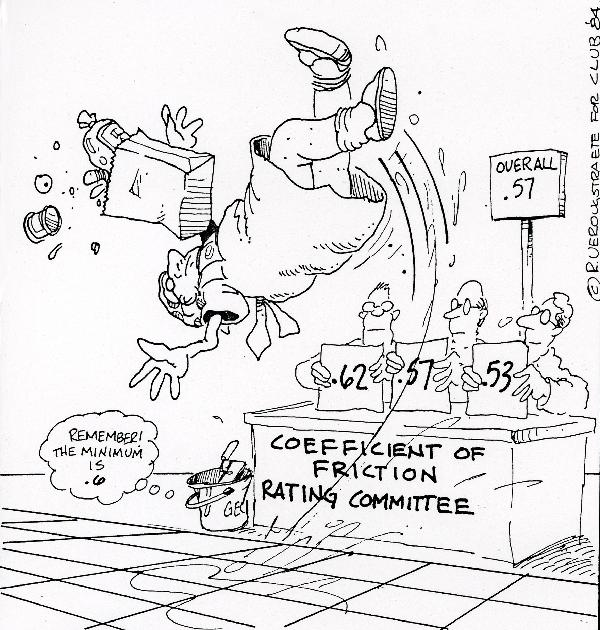Unit 05

Next UnitOverview: The focus of this unit is on the 2D motion of objects. Our studies thus far have focused on objects traveling in 1D motion (aka back and forth OR up and down). In this unit we will explore the nature of objects as they travel at angles, in circles, or along a trajectory!

Prior Knowledge: Previous Physics Units, Sine, Cosine, Tangent, Tangent Lines, Circumference, Properties of Right Triangles, Law of Cosines they travel at angles, in circles and along trajectories.

Useful Tips: Be sure to have a scientific calculator that has basic trigonometry functions,

Unit Documents

Unit Outline

Note Packet(s)

Presentations (N/A in this Unit)

Equations Sheet

Textbook Resources

Interactive Lesson(s)

Practice Test(s)